﻿ A3Mark: Seismic Attributes Benchmark

# A3Mark Datasets

A base 3D seismic cube was created, composed of different spectral components emulating a perfect sphere, and hence containing all possible dip angles, dip azimuths and curvature values. Moreover, we applied an anti-aliasing filter to cover steep dips. The base seismic cube we generated is useful if the users want to test robustness of their algorithms against borderline aliasing-situations at steep dips. It is also useful when one wants to test the vertical resolution of various tools.

Three tailored datasets were generated, covering various structural measurements. Each dataset contains a unique variant of the base 3D seismic cube with three levels of noise: noise free (Figure a1), 5dB random noise (Figure a2) and 5dB coherent noise (Figure a3).

Dataset 1 includes one normal fault (fault 1) and one reverse fault (fault 2), running parallel to the crossline axis, as shown in Figure b1. Another fault, fault 3, that intersects both fault 1 and fault 2 and is parallel to the inline axis is added in dataset 2, as seen in Figure b2. Intersecting faults add more challenges to geometrical attributes. Dataset 3 has one more fault, fault 4, which is not parallel to any of the axes, but intersects faults 1, 2 and 3, as illustrated in Figure b3; this increases the complexity.

Creating the Ground truth:

For each of our three datasets, four ground truths are computed analytically from each seismic cube, and are stored as separate cubes. They are defined as follows:

• Edge/Fault: The discontinuities (discrete values, 1 is edge/fault in yellow, 0 is non edge/fault in cyan), in Edge inline 40&116 Figure b.
• Dip angle: 3D tangent plane dip angle (continuous values ranging between [0°, 90°] in XYZ space, not IJK space), in Figure c1.
• Dip azimuth polar: 3D tangent plane azimuth angle, relative to polar north (continuous values ranging between [0°, 360°] in XYZ space, not IJK space), in Figure c2.
• Curvature K1: Principal curvature for current sample defined as 1/Radius (continuous values ranging between [-0.25, 0.25]), in Figure c3.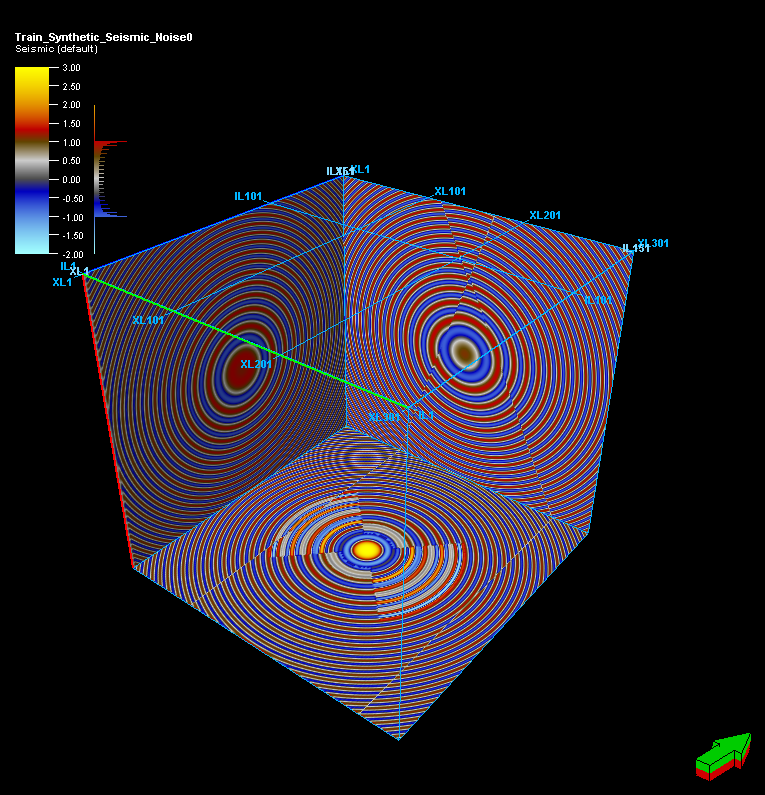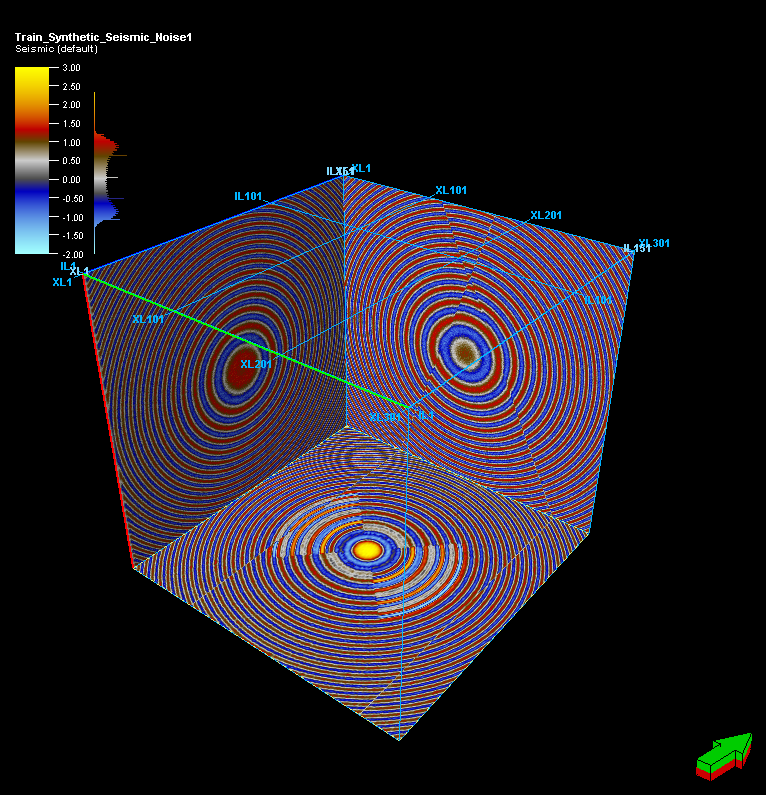(a1) Dataset 1, Seismic, noise free (a2) Dataset 1, Seismic, random noise (a3) Dataset 1, Seismic, cohenrent noise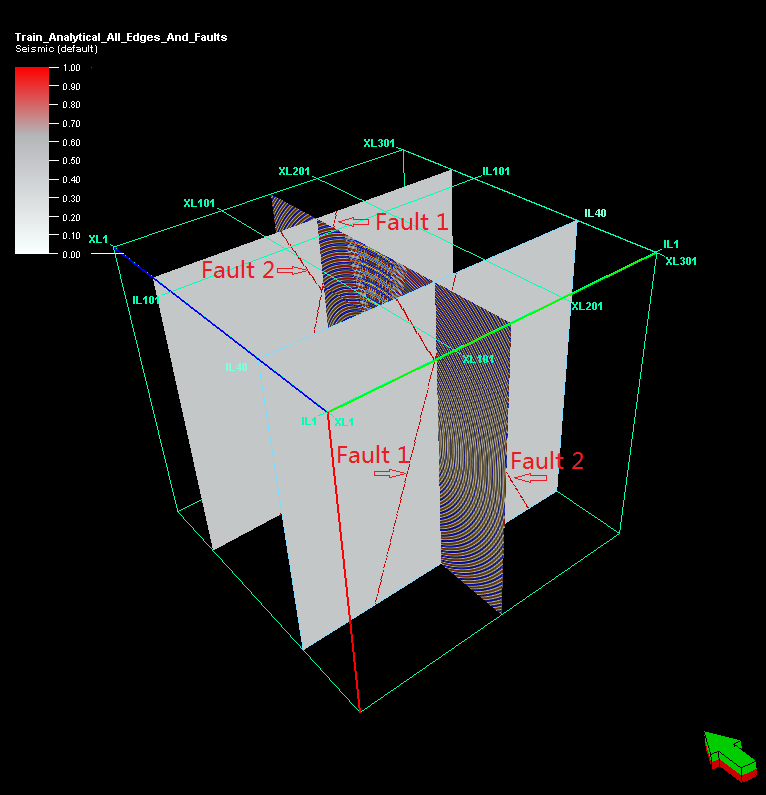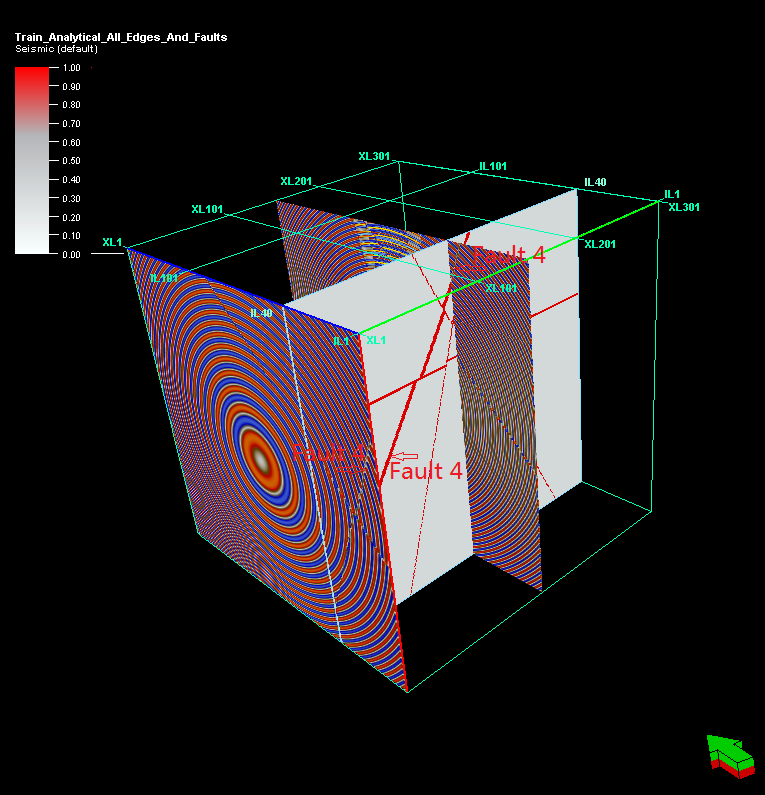(b1) Dataset 1, Edge, inline 40&116, Seismic, xline 151 (b2) Dataset 2, Edge, inline 40, xline, 1&151 (b3) Dataset 3, Edge inline 40, xline, 1&151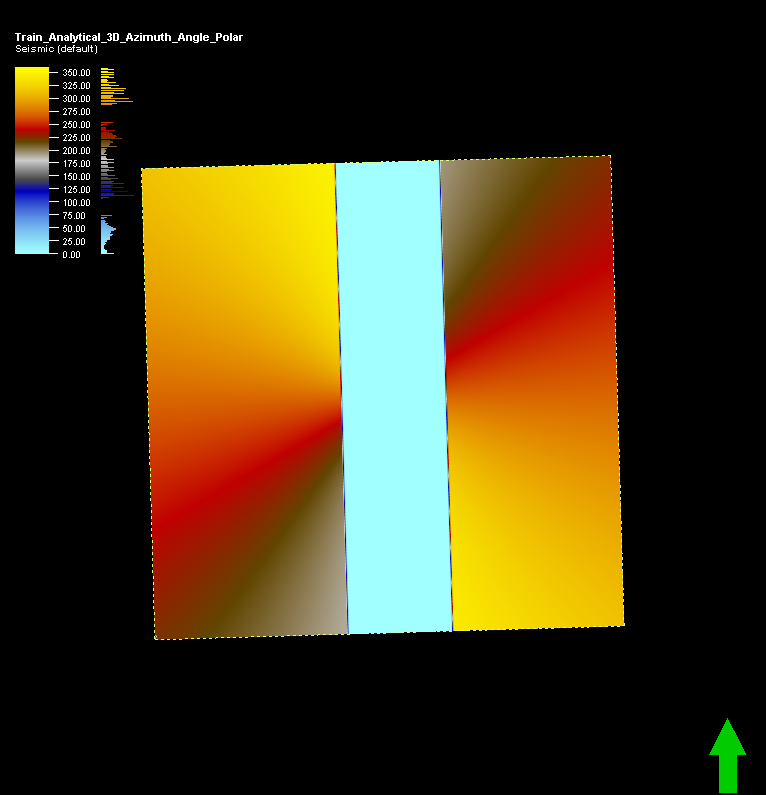(c1) Dataset 1, Dip angle, xline 151 (c2) Dataset 1, Dip azimuth polar, time -1800 (c3) Dataset 1, Curvature k1, inline 76 Figure: Snapshots of some seismic data and ground truth